# Experiment 1

The first experimental design tool test.

## Introduction

My initial plan in an ideal scenario would have been this:

1. figure out what sensor I need to determine 2 dimensional position (position sensor)
2. make a simple circuit with a switch (on/off button) that records the x and y coördinates
3. write processing code (or another language) that uses this x and y data to draw dots/lines (dots together form the line) when the button is pressed
4. add a sensor with an analogue output that records the loudness of surrounding noise
5. connect that value to the width/scatter of the dot (“brush”) > use increments so the line will be less bumpy
6. make different (scatter) brushes in processing
7. add buttons for different brushes

But this went south during the first step already as apparently determining 2 dimensional position (absolute or relative) is incredibly difficult. My first experiment will therefore be to figure out how I can use the sensor data generated by an accelerometer in a Processing sketch, and if I maybe can make another experimental design tool using that. I did get the suggestion from a friend to try positioning using a camera and OpenCV so I may look into that later.

But first: the start of the accelerometer experiment.

## Used sensors and items

• Accelerometer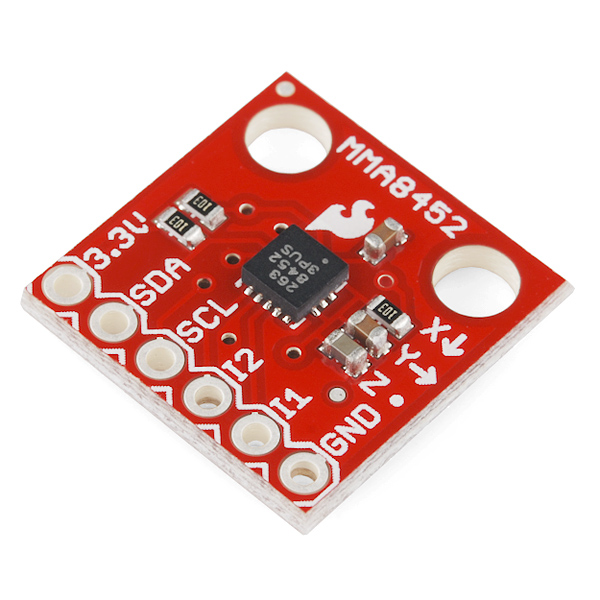Pin Label Pin Function Input/Output Notes
3.3V Power Supply Input Should be between 1.95 - 3.6V
SDA I2C Data Signal Bi-directional Bi-directional data line. Voltage should not exceed power supply (e.g. 3.3V).
SCL I2C Clock Signal Input Master-controlled clock signal. Voltage should not exceed power supply (e.g. 3.3V).
I2 Interrupt 2 Output Programmable interrupt — can indicate data ready, orientation change, tap, and more.
I1 Interrupt 1 Output Programmable interrupt — can indicate data ready, orientation change, tap, and more.
GND Ground Input 0V/common voltage.
• Jumper wires
• Arduino Uno
• 330Ω Resistors

## Goals

• To see how an accelerometer works
• To convert the x and y data to something visual using Processing and Arduino

## Important

The MMA8452Q has a maximum voltage of 3.6V – that range applies to both the power supply and the I2C pins. Because I am using an Arduino (5V), some level-shifting is required between devices.

### Step 1: soldering the headers to the breakout board

Make sure you follow these steps when soldering.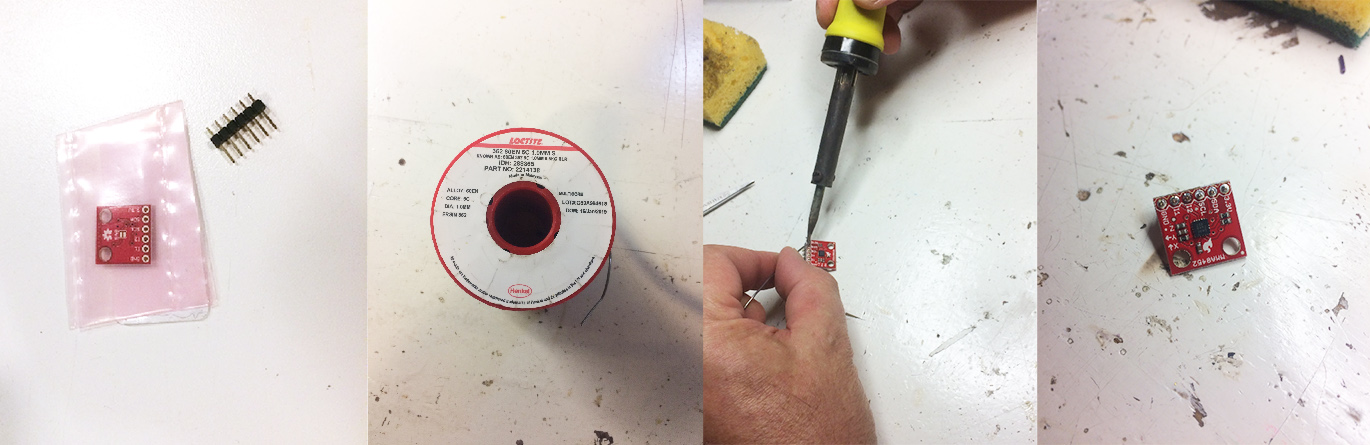### Step 2: setting up the board using the hookup guide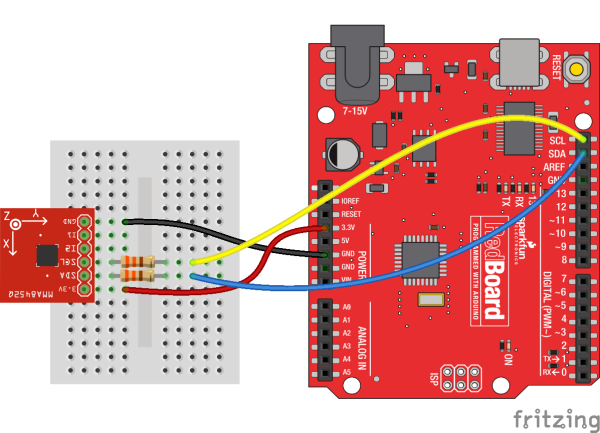Since the MMA8452Q’s maximum voltage is 3.6V, you’ll need to do some level shifting between your Arduino and accelerometer. Powering the accelerometer off the Arduino’s 3.3V rail is a good start, but you’ll also need to add some protection on the SDA and SCL lines.

In the example hookup above, we used a pair of series resistors on the SDA and SCL lines. This version of “level shifting” works in a pinch, but, if you want a more reliable level-shifting setup, we recommend using a more robust level shifter between the boards.

• Since there were no 330 ohm resistors available I put multiple resistors in series to create the same amount of resistance. Using a multimeter I measured the following resistance: 334.1 ohm on the SCL pin and 333.6 ohm on the SDA pin.
• I’m using an Arduino Uno, on which the A4 pin corresponds with the SDA pin and the A5 pin with the SCL pin.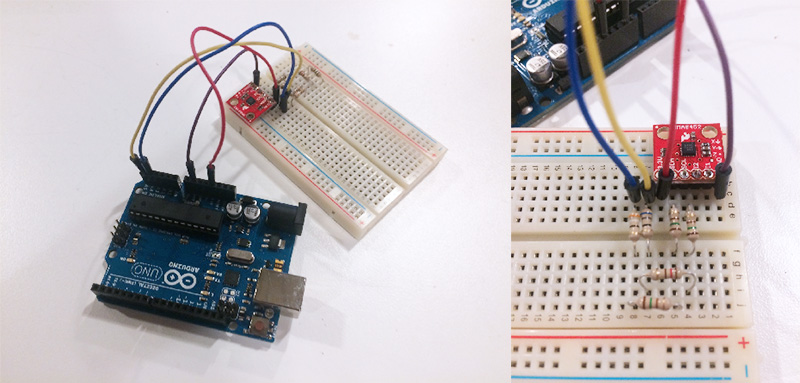### Step 3: test using the example code

The output result from the example code can either be in raw x, y and z data, or calculated acceleration values read out of the accelerometer; these are in units of g’s.

#### Raw data

However these values are not the kind of x, y and z data that I wanted; apparently it is very hard to determine position using sensors. What I can do is use this data for other purposes in my tools (or to figure out a new way of illustrating using these values) but that will not fix my current problem.

### Step 4: connecting Arduino to Processing to use the generated data

To accomplish this, I used the provided example code for the accelerometer provided by Sparkfun. I added some more comments for clarity. https://github.com/sparkfun/SparkFun_MMA8452Q_Arduino_Library/tree/master/examples/Example2_RawDataReading

### Processing code

This was based on the final answer on this forum: https://processing.org/discourse/beta/num_1254511350.html. The next step would be to figure out how to separate the raw data into separate arrays.

The standard mode is 2g mode, so the sensitivity is 1024 counts/g.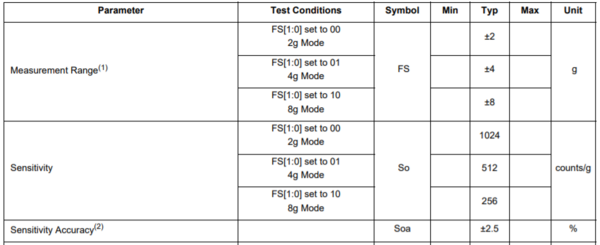When placed flat on the floor, these are the measured values: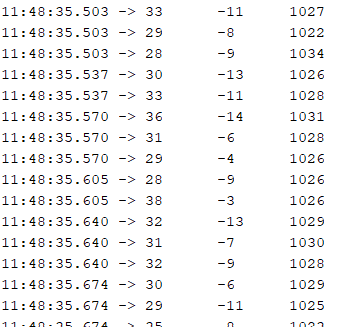### Step 5: Sensor data into arrays

To use the data in Processing, you have to read the entire input line from the Arduino, then split the line with a comma (then you have a String[]) and parse each number into int[] with index one to one matching.

### Step 6: Use array data to generate art

The video above was my first try at using the accelerometer values. To draw with the accelerometer, I wrote the following code underneath println() in the code above.

This is the result after a bit of trying out: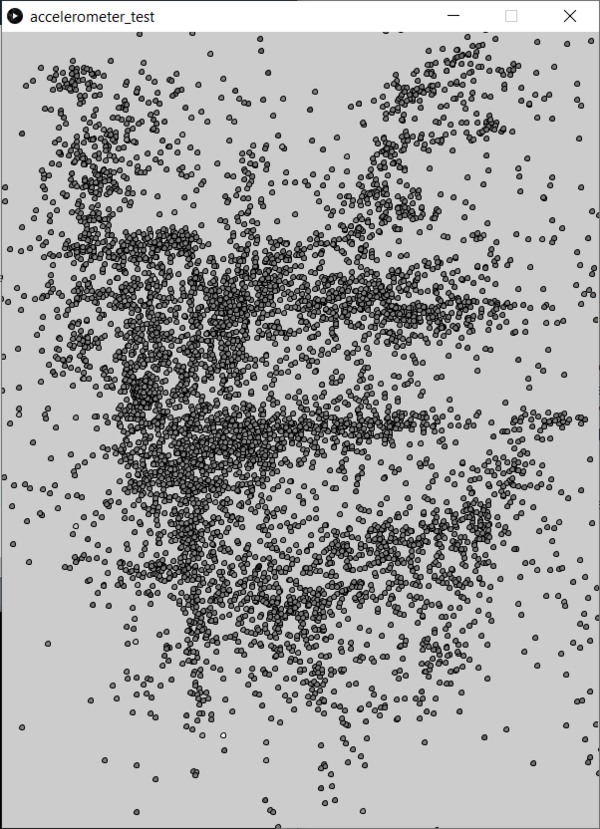After this I worked on modifying the Processing code to make the illustration better. I also used a higher baud rate to receive more data so I would be able to make smoother lines.